﻿ Difference Delay Equation-Based Analytical Model of Hematopoiesis
Journal of Biomedical Systems & Emerging Technologies
All submissions of the EM system will be redirected to Online Manuscript Submission System. Authors are requested to submit articles directly to Online Manuscript Submission System of respective journal.
• Research Article
• APSF 2012, Vol 1(1): 102

Difference Delay Equation-Based Analytical Model of Hematopoiesis

Probir Kumar Dhar1,3, Abhik Mukherjee2 and Durjoy Majumder1*
1Department of Physiology, West Bengal State University, Berunanpukuria, Malikapur, Barasat, North 24 Pgs., Kolkata 700 126, West Bengal, India
2Department of Computer Science and Technology, Bengal Engineering and Science University, Shibpur, Botanic Garden, Howrah 711 103, West Bengal, India
3Department of Electronics and Communication Engineering, Bengal College of Engineering and Technology, Shahid Sukumar Banerjee Sarani, Durgapur 713 212, West Bengal, India
*Corresponding Author: Durjoy Majumder, Department of Physiology, West Bengal State University, Berunanpukuria, Malikapur, Barasat, North 24 Pgs., Kolkata 700 126, West Bengal, India, Email: [email protected]

Received Date: Aug 15, 2011 / Accepted Date: Dec 26, 2011 / Published Date: Dec 28, 2011

Abstract

Hematopoiesis is a complex dynamic process by which all the blood cells of the peripheral blood are formed. Different molecular techniques identified different molecules involved in a coordinated and time-dependent fashion for the development of different blood cells of specific lineages. These are identified with the cells in isolation that may obscure the dynamical nature of hematopoiesis. This has an importance in understanding of different hematological diseases. However, there is no generalized model available in the literature by which clinicians and patients can be benefited. We have developed a systems model based on difference delay equation. Our model shows that minor variation as well as incorporation of a minor stochastic component to that model equation at the morphological stage(s) may exhibit different pathophysiological state of hematopoietic system. Therefore, this mathematical model reinforces the necessity of expert domain knowledge in translating the parametric values so that individual patient could be benefited.

Keywords: hematopoiesis; difference delay equation; leukemia

Introduction

Hematopoiesis is a complex process of blood cells development from hematopoietic stem cell. Hematopoietic stem cells undergo through the stages of differentiation processes to form progenitor cells of different lineages. These progenitor cells undergo further maturation stages to form mature cells, namely erythrocytes (RBCs), leukocytes (WBCs) and thrombocytes (THBs) (platelets) of the hematopoietic system. Different biomolecules, namely cytokines, cell signaling molecules and transcription factors, synergistically act to control the differentiation processes of this cellular development . Any alteration of these molecules may lead to the development of hematological diseases (HDs). Phenotypes of differentiated lineages reflect the sets of genes expressed therein as well as the receiving signals by the cytokines, therefore the black box modeling could have an implication in the understanding of hematopoietic dynamics . Under normal condition, the cytokine erythropoietin (EPO) regulates the erythrocyte production by increasing the production of primitive erythroid precursors and finally an increase occurs in the number of circulating erythrocytes that take place some days later [1,21]. In the production of white blood cells, granulocyte colony stimulating factor (G-CSF) has been demonstrated to be very important . Thrombopoietin (TPO) regulates platelet production by controlling the production and maturation of megakaryocytes. These are platelet precursor cells that give rise to 1000–5000 platelets each [11,20,22].

Previously, several mathematical models of hematopoiesis have been proposed. Different authors substantially identified different controlling variables whose alteration leads to the manifestation of HD. It has been shown that oscillations and delay in the stem cell compartment are important and drive several periodic HD [9,14,17] such as cyclical neutropenia (CN), periodic chronic myelogenous leukemia (PCML), cyclical thrombocytopenia, and periodic hemolytic anemia. CN and PCML are of special interest as oscillations in all three cell lines occurring within the same oscillation period . Using G0 stem cell model, it was possible to explore the oscillations at the neutrophil level but not at the platelet level. Using integrated mathematical model, it was possible to duplicate various features of CN. These have been addressed in the unified model of PCML and could be fitted well with the insertion of maturation delay time rather than only in the proliferation phase of a single discrete cell line [4,5]. Based on the simulations, authors substantially argued that the critical model parameters include the amplification rate in the leukocyte line, the differentiation rate from the stem cell compartment into the leukocytic lineage and the rate of apoptosis in the stem cell compartment. By using differential delay equation, it has been shown that oscillation in CN is due to the slow periodic oscillations in the stem cell level .With a decoupled model of stem cell, addition of stochastic perturbation with noise term(s) at the differentiation/multiplication or apoptosis rate of stem cell level, it was also possible to introduce fluctuations in the cell number .

A four-compartment mathematical model has been developed by Michor et al. to understand the treatment dynamics of chronic myeloid leukemia . RQ-PCRbased data (of BCR-ABL) points have been fitted to the mathematical model for the evaluation of developing imatinib resistance mutation. In this approach, quantitative evaluations as well as numerical estimates of the turn-over rates of leukemic progenitors and differentiated cells are possible. Authors also concluded that the treatment failure is due to harboring of resistance mutations increase with disease progression as a consequence of increased stem cell burden, and multiple drug therapy is suggested to be especially important for the patients who were diagnosed with advanced and rapidly growing disease.

However, the models developed earlier are based on data that are difficult to get and its translation into a clinical scenario is practically impossible. In recent times, different biological parametric estimation procedures including the RQPCR have been criticized by a section of the scientific community . Firstly, the limitation is at the instrument level, as the majority of the instruments have the reliability of data within 15% of coefficient of variation (CV) . Secondly, there is a large variation of a particular parameter even at the smaller population level and a very small perturbation at the initial factor may produce a large drift during a given time course . Therefore, for prediction of a disease dynamics, it may not be possible to apply the population level data to the individual cases. Hence, for clinical applications or handling of different clinical problems of hematology, an easy-to-handle analytical model is required, so that clinicians can easily fit the clinical data that are particularly captured in a cost-effective manner to that analytical model for getting a prediction regarding the individual clinical cases. The present work tries to develop an analytical model in that direction. To achieve this, we have used the existing knowledge of hematopoietic dynamics at the morphological level together with the different functional characteristics of the hematopoietic cells.

Methods

Hematopoiesis model formulation

Like others we have also assumed the three lineages model of hematopoiesis. The model has been schematically presented in Figure 1. In the model, it is assumed that stem cell(s) is/are differentiated into the precursor cells of three lineages, namely erythroid (p1), leukocytoid (p2) and megakaryocytoid (p3) lines. Precursor cells of each lineage are then differentiated into the corresponding mature cells— RBC (b1), WBC (b2) and thrombocyte (THB) or platelet (b3). For simplification of the model, all sorts of precursor cells of each of the lineages are being considered as only progenitor/precursor cells. Thus, this sort of model is a unidirectional model as described by others [4,5,16] and stem cells are being coupled with the cells of the peripheral blood. However, in contrast to the previous models, all parametric variables for simulation purposes are taken as the absolute cell numbers per cubic millimeter, the unit used in the conventional Neubauer hemocytometre chamber or by the cell counter. For leukocytic lineage we have considered the whole lineage instead of only neutrophil lineage [5,16]. The considered parameters of the model have been shown in Table 1.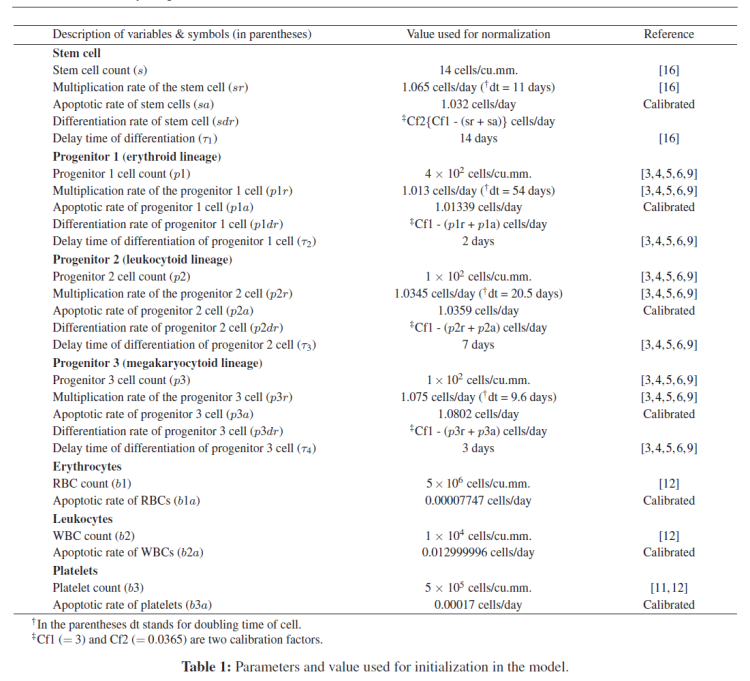System equation

The dynamical equation of the model is based on the difference delay equation. The system equation of cells of any maturation stage can be represented through the following generalized equation: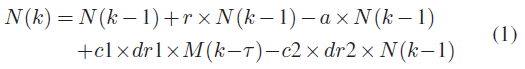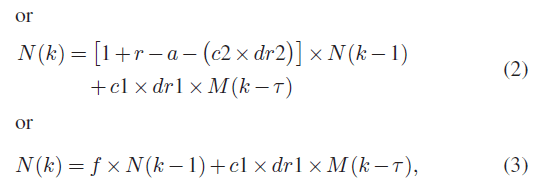where N(k) is the number of concerned cell type at time instant k and M(k−τ ) is the number of precursor cell type which was differentiated τ time ago. Hence, τ is the delay time required for cell maturation by the action of different cytokines, cellular signaling molecules and transcription factors. Each cells type grows exponentially with its own multiplication rate (r), and decays with its own apoptosis rate (a). Thus, for stem cell these variables are denoted with sr and sa. Similarly, for p1 lineage cells, these variables are represented by p1r and p1a. This notational scheme is followed for other cell types as represented in Table 1. It is assumed that multiplication rate of each cell type at the matured cells compartment is zero.

When the effect of differentiation is absent, that is, when k is less than the delay time (τ) required for the formation of the cell type of the down-stream lineage, c1 will be equal to zero. Otherwise c1 will be a positive quantity. In the present model, during the stage of differentiation from stem cell to progenitor cell stage, value of c1 will be equal to one divided by three (as stem cell is distributed to three lineages after differentiation). However, in the system equation for the cells of the subsequent compartment, c1 is equal to one (as each progenitor cell type is differentiated into a single lineage only). For the cells which are differentiable such as stem cell (s), erythroid (p1), leukocytoid (p2) and megakaryocytoid (p3), they will have c2 equal to one in their system equation. For the system equation of RBC, WBC and platelet, c2 will be equal to zero as they do not differentiate.

Again dr1 is the differentiation rate of the previous compartment cell and dr2 is the differentiation rate of the concerned cell type. For example, the differentiation of stem cell to the progenitor cells has only dr2 (as dr1 is zero) and is represented by sdr. Similarly, for p1 lineage cell type, dr1 = sdr and dr2 = p1dr. Likewise, for p2 lineage cell type, dr1 = sdr and dr2 = p2dr and for p3 lineage, dr1 = sdr and dr2 = p3dr.

Now, if k < τ (delay time required for the formation of a progenitor cell from its previous compartment cell of the same lineage) the system equation of concerned daughter cell types can be represented through the following generalized equation: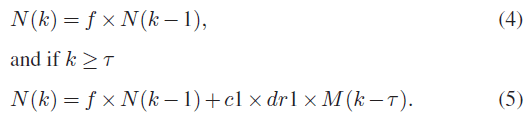Considering all the cell types and their corresponding functional factors, the above-mentioned generalized equation may be used to develop a system model. Again, the value of f is different for different cell types: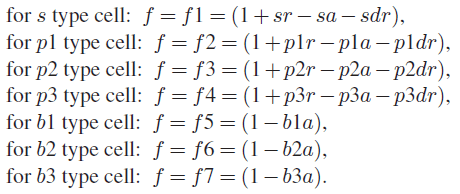In our system model, four delay times τ1, τ2, τ3, τ4 have been considered. Where τ1 is the required delay time for the formation of p1, p2 and p3. τ2 is the required delay time for the formation of RBC from p1. τ3 is the required delay time required for the formation of WBC from p2 and τ4 is the required delay time for the formation of platelets from p3. Let us assume τ1 <τ2 <τ3 <τ4 (note that in the model there is no such hierarchy and the model is flexible in nature).

At discrete time interval (k), the overall system equation can be written as when k <τ1,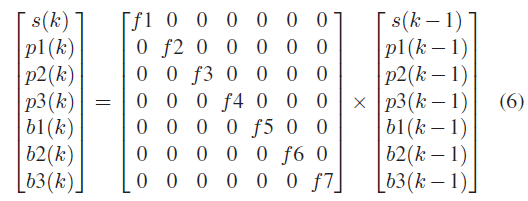or x(k) = Ax(k − 1), where x(k) = [s(k),p1(k),p2(k), p3(k),b1(k),b2(k),b3(k)]T, A = Diag[(fi) 1 ≤ i ≤ 7] matrix given in (6).

For kτ1, the equation (6) is changed as follows: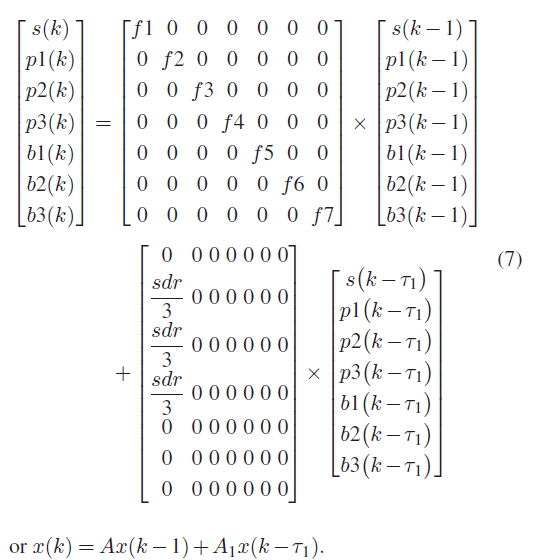For kτ2, the equation (7) is changed as follows: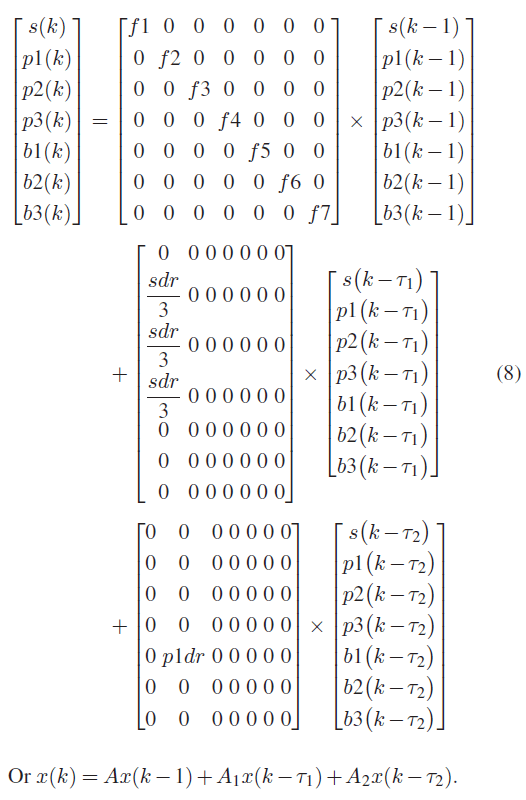For kτ3, the equation (8) is changed as follows: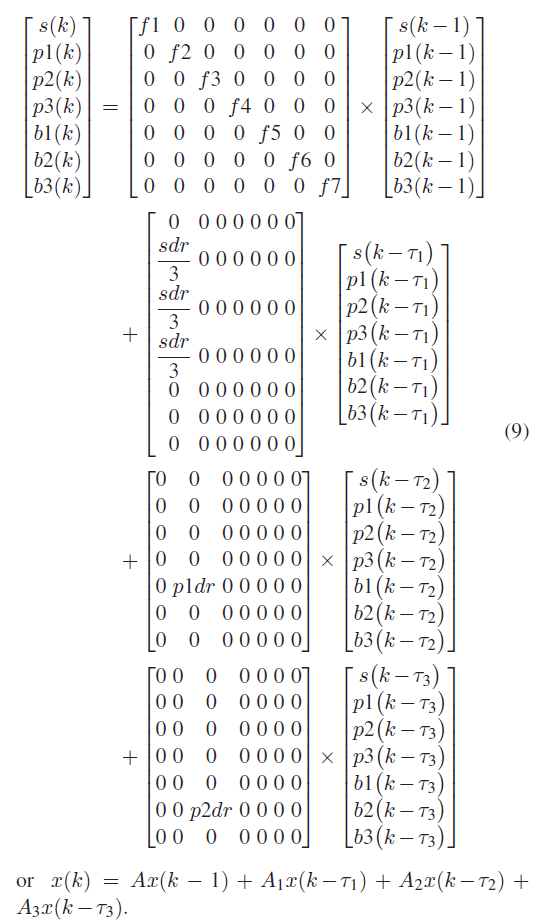For kτ4, the equation (9) is changed as follows: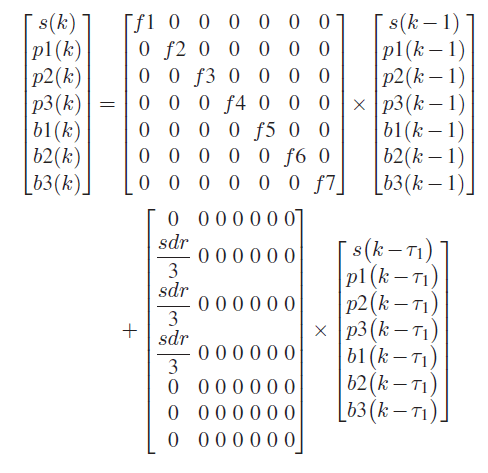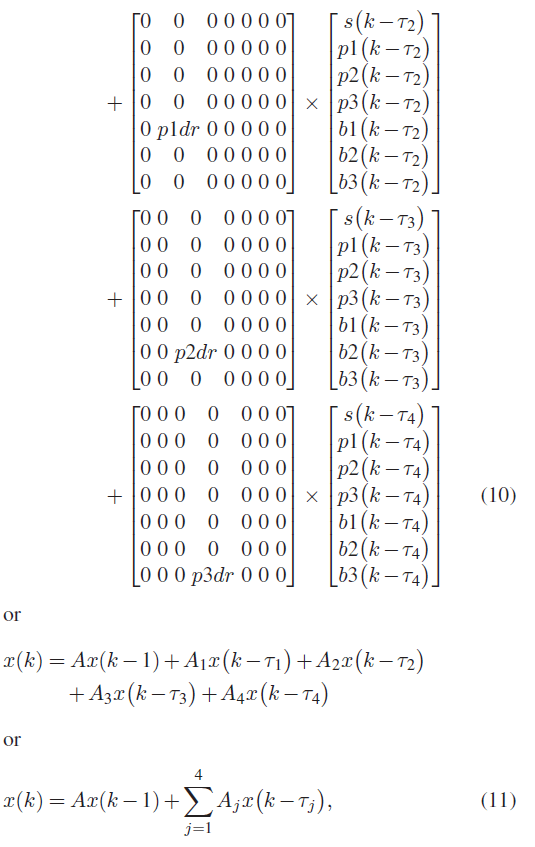where A and Aj both are defined by (7×7) matrix.

Simulation Results

With the previously mentioned systems equations, simulation exercises have been carried out using MATLAB 6.5. The parametric values as mentioned in Table 1 are utilized for rationalizing the dynamical states of the hematopoietic system and to understand the relative importance of those parametric values in hematopoietic dynamics.

Settings of variables for the hematopoiesis

The values have been set by considering the hematopoietic process for a normal individual and are presented in Table 1. The values of cell numbers are presented in terms of absolute cell number per cubic millimeter and multiplication rate (doubling time), differentiation rate and apoptosis rate are all in number of cells per days, while time delay in differentiation is in number of days. For setting of parametric values we used the references [3,4,5,6,9,11,12,16]. In majority of the cases total cell count data are available, functional data in most of the cases are calibrated data. Parametric values of the peripheral blood are obtained from references [11,12], stem cell level data are collected from the work of Michor et al. 2005 . Different parametric values for the variables of the progenitor cell level for human (normal) are not available in literature, however, dog model data in terms of body weight are available [3,4,5,6,9]. So for fitting into the model we make a logical transformation of that data in terms of cu.mm. with some calibrations to fit into the model.

Simulation studies show that with the setting of these initial parametric values as mentioned in Table 1, the absolute cell number of different progenitor and mature cells in the peripheral blood are maintained very closely to the initially set value (Figure 2).

Figure 2: Dynamics of immature progenitor cells (a) and mature cells (b) for a normal individual as simulated with the parametric values mentioned in Table 1. In the plot “Total” indicates the total number of s-type, p1-type, p2-type and p3-type cells (a), and RBCs, WBCs and thrombocytes (THBs) or platelets (b).

Identification of the sensitive parameter

It is acknowledged that in different hematological disorders, the parametric value changes. To identify the sensitive parameter and the impact of that parameter in the development of hematological disorder in the long run, simulation study would be helpful. Hence, it is necessary to identify the influence of each parameter in the dynamics of the hematopoiesis. It is also acknowledged that in several hematological disorders, different combinations of parameters may be altered. When diseases are identified, there is a large deviation of the parametric mean in the diseased person in comparison to the normal. However, it is not known whether or not the minor variation in the value of different hematological parameters and their persistence within the system would influence the hematopoietic system behavior even though the values are within the normal population variance. In that case, analytical model and simulation study may also help.

To test the systems sensitivity and influence of the parametric values on the manifestation of different hematological disorders, rigorous simulation exercises have been carried out. The values of the variables have been changed within 10–100% of the setting values. Simulation results suggest that the change in parametric values of peripheral blood compartment has almost no impact on the dynamics of the overall system. On the other hand, minor variations, even within the 10% of the mean value of any parameter in the bone marrow (immature cells) compartment, have a considerable impact on the overall system dynamics. In this compartment, multiplication rate, apoptotic rate and differentiation rate have much influence on the systems dynamics within a shorter time period, whereas differentiation delay has influence for the longer time period. For example, if differentiation rate in the erythroid lineage is reduced to 1/6 (from s to p1) and 1/60 (from p1 to b1) of the initial set value as shown in Table 1, and keeping other parametric values as mentioned in Table 1 unchanged, there is much effect on the progenitor compartment rather than in the peripheral blood (Figure 3). However, decreasing the number of RBCs in the peripheral blood requires the increment of apoptosis rate of RBC. From this simulation, it is anticipated that the increase in apoptosis rate may be a major factor for the development of anemia in acute condition. However, the decrease in differentiation rate may produce a chronic effect in the development of anemia.

Figure 3: Simulation result with the change in the parametric values sdr ≅ 0.0055 and p1dr ≅ 0.0162 keeping other parametric values unchanged as mentioned in Table 1. This simulation resembles the anemic feature. In (a), cells of the immature progenitor compartment and in (b) cells of the mature cells compartment are shown. In the plot “Total” indicates the total number of s-type, p1-type, p2-type and p3-type cells (a), and RBCs, WBCs and thrombocytes (THBs) or platelets (b).

With the increase in the multiplication rate and differentiation rate, the system response becomes unbounded in nature; while the decrease in the apoptotic rate (keeping the parametric values of other variables unaltered) simulation studies show the same behavior. With the reverse condition, systems may tend to collapse. However, the absolute cell number of any compartment does not have any influence on the qualitative behavior in the system dynamics though the output has an increased number of cells. Change in the parametric value at the progenitor compartment has the influence on that particular lineage. With increase in multiplication rate of p2r to 1.035 and further decrease in p2dr to the 1/10 of the calculated value according to the relationship mentioned in Table 1 and keeping other parametric values as mentioned in Table 1 unchanged, hematopoietic dynamics resembles the behavior with leukemic (Figure 4).

Figure 4: Simulation result with the change in the parametric values p2r = 1.035 and p2dr ≅ 0.093 keeping other parametric values unchanged as mentioned in Table 1. This simulation resembles the leukemic feature, as there is an increase in the immature progenitor cells of leukocytic lineage (a) with the decrease in the mature WBCs (c), other cells in peripheral blood (b), is almost unchanged. In the plot “Total” indicates the total number of s-type, p1- type, p2-type and p3-type cells (a), and RBCs, WBCs and thrombocytes (THBs) or platelets (b).

Stochastic perturbation

Any sort of biological observation is noisy and has been represented as the mean value with a variance. This is due to three reasons: first, the non-homogeneous nature of the biological sample; second, the variation due to the detection system; third, the experimental error. Conventional clinical testing consisting of collected data at a discrete sequence of time may show a large deviation of the parametric value from its mean value within two consecutive time points. Loss of such consecutive data may influence the systems output in the long run, if a dynamical model is set with the population mean value. Incorporation of minor stochastic component within standard deviation of the mean value of the parametric variable(s) gives the simulation study a more realistic flavor.

Moreover, the change in the parametric value of the peripheral blood compartment has no qualitative influence on the system dynamics. Hence, it is important to study the effect of stochastic perturbation with the parameters of the cells present in the bone marrow compartment. The following simulation study reveals the parametric influence at the stem cell level. Stochastic component is introduced in the developed model as an additive term to the sr; hence, the parametric value of sr will be changed to srm. Hence,

srm = sr+mf×rand,

where rand is a function for generating random numbers. This has been done through MATLAB. mf is a multiplication factor. With the addition of this stochastic component around the mean value of multiplication rate (sr = 1.065) at every time interval (Table 2) while keeping other parametric values as mentioned in Table 1 unchanged, a change in the system dynamics is noted. Simulation result shows that with the increase in the standard deviation around the mean value of the multiplication rate (sr), there is a more deviation in the system output from the normalized condition within a given interval of time (Figures 5 and 6). This is noted particularly in the bone marrow compartment. Interestingly, with the same sr and mf values (the value used for the simulation of Figure 5), but due to the presence of stochastic component another simulation exhibit another pathophysiological state that is resemble with the pancytopenic characteristics of the hematopoietic dynamics (Figure 7).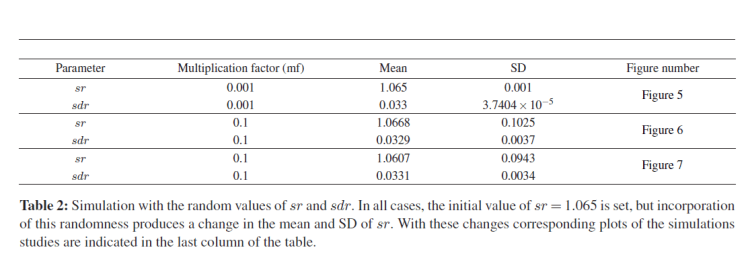Figure 5: Profile of the dynamics of immature progenitor cells (a) and mature cells (b) with a random value and an initial set value of sr = 1.065 and mf = 0.001 while other parametric values, as mentioned in Table 1, were kept unchanged. But due to the addition of stochasticity, the mean value of sr is changed to 1.065. In the plot “Total” indicates the total number of s-type, p1-type, p2-type and p3-type cells (a), and RBCs, WBCs and thrombocytes (THBs) or platelets (b).

Figure 6: Profile of the dynamics of immature progenitor cells (a) and mature cells (b) with a random value and an initial set value of sr = 1.065 and mf = 0.1 while other parametric values, as mentioned in Table 1, were kept unchanged. But due to the addition of stochasticity, the mean value of sr is changed to 1.0668. In the plot “Total” indicates the total number of s-type, p1-type, p2-type and p3-type cells (a), and RBCs, WBCs and thrombocytes (THBs) or platelets (b).

Figure 7: Profile of the dynamics of immature progenitor cells (a) and mature cells (b) with a random value and an initial set value of sr = 1.065 and mf = 0.001 while other parametric values, as mentioned in Table 1, were kept unchanged. But due to the addition of stochasticity, the mean value of sr is changed to 1.0607. In the plot “Total” indicates the total number of s-type, p1-type, p2-type and p3-type cells (a).

Discussion

Simulation studies with this analytical model suggest that the persistence of one of the parametric values with a minor shift is able to produce pathophysiological shifting in the hematopoietic system. Our simulation study indicates that the occurrence of different hematological disorders may be due to the minor shifting of parametric value particularly at the immature cell stage. In reality, it is not known whether that value may persist for longer interval of time for the manifestation of the disease phenotype. Moreover, due to the large variation of biological data at the individual level and instrumental limitation, it is difficult to make a long-term prediction regarding the individual clinical cases with the population mean value. Simulation study with incorporation of stochastic component at the more immature cell stage also indicates the chances of appearing of a disease phenotype. Therefore, this study emphasizes the importance of precise data collection particularly at the immature cell stage during a clinical investigation procedure, as an imprecise data collection may increase the chance of erroneous prediction about the system dynamics. This sort of uncertainty can be minimized by increasing the amount of data collection in a given interval of time. Together with this, there is a need for the development of instruments having higher sensitivity. However, both approaches may increase the therapeutic cost. Cost can be minimized by observing the data for a shorter period of time and then, fitting it to the mathematical model with a proper calibration factor to predict the system dynamics.

Experimentally stem cells number, multiplication rate, apoptosis rate can be measured but, realistically there is no direct way to measure the differentiation rate of cells particularly for in vivo. At present moment acquisition of data for cell count at different lineages can be captured by flow cytometric analysis. For the multiplication rate, apoptotic rate and differentiation rate and delay time for cellular differentiation, the best way is through in vitro cell/bone marrow culture-based method with video documentation. Number of quiescent cells can be calculated by subtracting the sum of numbers of the apoptotic cells, multiplied cells and differentiated cells from the total number of cells at any time point. Thus, the number of the quiescent stem cells as described earlier  can be obtained. The advantage of such a dynamical model is that the absolute number of total progenitor cells could be evaluated at any time point, once the dynamics is fitted with this analytical model and then, prediction about the systems dynamics can be made. For implementation purpose, data obtained at the time of diagnosis can be fitted into this analytical model and thus future predictions can be made through simulation exercises. In this way, our analytical model would help the clinical investigators in the assessment regarding the future disease states of the patients.

An analytical model can infer regarding the importance of the range of parametric bounds in the prediction of systems dynamics. For many years physiologists and clinical investigators try to understand the importance of the initial parametric values in the prediction of systems dynamics but were unable due to lack of proper analytical tool. Attempt to finding this experimentally would definitely increase the cost of investigation. So they are speculative regarding this manner. It is hypothesized that in the application of Systems Biology (SB) to the clinical arena, middle-out rationalist approach (MORA) is more desirable than the conventional practices of SB. In the practice of MORA view, a set of difference equations with inequations are better suited for the quantitative understanding regarding the disease dynamics. This approach has already been applied for the development of analytical models of different pathophysiological states of solid tumors . The present model is based on difference delay equations. The incorporations of different delays in the system equation may be another representation of application of inequations. Due to difference equation, this analytical model has flexibility to change its parametric values and to incorporate other variable(s), if necessary, depending on the need of the individual clinical cases. This, in turn, helps to visualize/test the effect of that component(s) on the system dynamics and/or enhance the understanding of the complexity of a physiological system further. For example, the effect of interleukin on the hematopoietic dynamics may be observed by incorporating it as a subtractive or additive term into the present system equation. The expected out-come from this analytical model or further refinement of the model (by finer adjustments of parametric values or incorporation of calibration factor or other variables, if needed) can be achieved through the steps of predict-observe-correct cycle. Previously, this has been indicated as the implicative procedure for the developed (analytical) models through MORA view .

Therapeutic strategies can also be assessed through this model. Any drug which may alter these factors can bring changes in the dynamics in the long run. So the application of a specific drug (therapy) for a shorter interval of time, followed by collection of individual patients’ data and fitting of those data to the model followed by simulation exercises, can help to judge the efficacy of that particular drug in the long run. Therefore, through this model, hematologists can make predictions and assessment regarding the therapeutic outcome of a therapeutic schedule/strategy during the course of a treatment procedure.

A change in any molecular factor like cytokine level, signaling protein or transcription factor, would ultimately make a shift in the cellular functional characteristics, that is, multiplication, differentiation or apoptosis rates. This study also indicates the fact that with the available instrumental facility it is very difficult to translate a low level (molecular) data for prediction at the gross morphological characteristics or functionality of cells. In disease cases, a change in the subtractive terms or multiplication and/or differentiation terms may produce control. As a suggested strategy for the development of analytical model with MORA view, the presently developed model is based on the pathophysiological rationality and it considered hematopoiesis as a dynamical event. Hence, prior domain knowledge has been required to be the important criteria for understanding the implication, further development and/or modification of such analytical model . And we hope that this analytical model would help the physiologists and clinical investigators in testing their apprehensions and hypotheses regarding the patho-physiological shifting during the course of hematopoietic dynamics.

References

Select your language of interest to view the total content in your interested language

Recommended Journals
Viewmore
Article Usage
• Total views: 11546
• [From(publication date): 12-2012 - Jun 20, 2019]
• Breakdown by view type
• HTML page views: 7806Can't read the image? click here to refresh